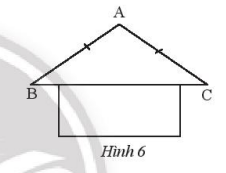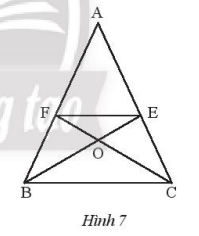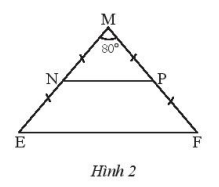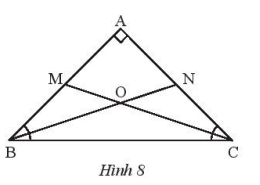## SBT Solution Lesson 3: Isosceles Triangle (C8 SBT Math 7 Horizons) – Math Book

SBT Solution Lesson 3: Isosceles triangle (C8 SBT Math 7 Horizon)
============

### Solve problem 1 page 49 SBT Math 7 Creative horizon episode 2 – CTST

Given triangle MNP is isosceles at M. Name the sides, base, vertex angle, and base angle of that isosceles triangle.

Detailed instructions for solving Lesson 1

Solution method

Use the definition of an isosceles triangle to name the sides, base, top angle, and base angle.

Detailed explanation

Triangle MNP bound at M has: side sides MN and MP, base side NP, top angle $$\widehat M$$, base angle $$\widehat N$$ and $$\widehat P$$

–>

— *****

### Solve problem 2 page 49 SBT Math 7 Creative horizon episode 2 – CTST

a) Is a triangle with two angles equal to $${60^o}$$ a triangle or not? Find the remaining angle of this triangle.

b) Is a triangle with two angles equal to $${45^o}$$ an isosceles triangle or not? Find the remaining angles of this triangle.

Detailed instructions for solving Lesson 2

Solution method

Use the definition of an isosceles triangle.

– Use the theorem that the sum of the three angles in a triangle is equal to $${180^o}$$ to calculate the remaining angles of the triangle.

Detailed explanation

a) The remaining angle is equal to: $${180^o} – {2.60^o} = {60^o}$$. This triangle is both an equilateral triangle and an isosceles triangle at its three vertices.

b) The remaining angle is equal to: $${180^o} – {2.45^o} = {90^o}$$. This triangle is both an isosceles triangle and a right triangle and is called an isosceles right triangle for short.

–>

— *****

### Solution 3 page 49 SBT Math 7 Creative horizon episode 2 – CTST

In Figure 6, calculate angle B and angle C knowing $$\widehat {{A^{}}} = {138^o}$$Detailed instructions for solving Lesson 3

Solution method

Use the isosceles triangle property to find the measure of the angle you want to find

Detailed explanation

We have: $$\widehat B = \widehat C = \frac{{{{180}^o} – {{80}^o}}}{2} = {50^o}$$(because of triangle ABC weigh at A)

–>

— *****

### Solution 4 page 49 SBT Math 7 Creative horizon episode 2 – CTSTGiven figure 7, know AB = AC and BE is the bisector of $$\widehat {ABC}$$, CF is the bisector of angle $$\widehat {ACB}$$. Prove that:

a) $$\Delta ABE = \Delta ACF$$

b) Isosceles triangle OEF

Detailed instructions for solving Lesson 4

Solution method

– Use the property of bisectors to show that two angles are congruent from proving two triangles are congruent.

– Proving OE = OF should deduce triangle OEF isosceles

Detailed explanation

a) we have AB = AC, so triangle ABC is isosceles at A, so $$\widehat B = \widehat C$$

Otherwise: $$\widehat {FCA} = \frac{{\widehat C}}{2}$$ (because CF is the bisector of angle $$\widehat {ACB}$$)

$$\widehat {EBA} = \frac{{\widehat B}}{2}$$ (because BE is the bisector of $$\widehat {ABC}$$)

So $$\widehat {FCA} = \widehat {EBA}$$

Consider triangle ACF and triangle ABE with:

$$\widehat {{A^{}}}$$general

AC = AB

$$\widehat {FCA} = \widehat {EBA}$$

Reason: $$\Delta ACF = \Delta ABE(g – c – g)$$

b) We have: $$\Delta ACF = \Delta ABE(g – c – g)$$ infer: BE = CF (1)

We have an isosceles triangle OBC at O, so OB = OC (2)

From (1) and (2) deduce BE – OB = CF – OC, so OE = OF

So triangle OEF is isosceles at O.

–>

— *****

### Solution 5 page 49 SBT Math 7 Creative horizon episode 2 – CTST

Given triangle MEF isosceles at M, there are $$\widehat M = {80^o}$$

a) Calculate $$\widehat E{,^{}}\widehat F$$

b) Let N, P be the midpoints of ME, MF, respectively. Prove that triangle MNP is isosceles.

c) Prove that NP // EF

Detailed instructions for solving Lesson 5

Solution method

Use the isosceles triangle property to find the measures of the angles

– Prove that MN = MP deduce the triangle MNP is isosceles at M

– Prove two angles $$\widehat {MNP} = \widehat {{\rm{NEF}}}$$ infer NP // EF

Detailed explanationa) Since the triangle MEF is isosceles at M, $$\widehat E = \widehat F = \frac{{{{180}^o} – {{80}^o}}{2} = {50^o}$$

b) We have an isosceles triangle MEF at M so ME = MF.

Derive: $$MN = \frac{{ME}}{2} = \frac{{MF}}{2} = MP$$

So triangle MNP is isosceles at M.

c) In isosceles triangle MNP we have: $$\widehat N = \widehat P = \frac{{{{180}^o} – {{80}^o}}}{2} = {50^o}$$

so $$\widehat {MNP} = \widehat {{\rm{NEF}}} = {50^o}$$

Derive NP // EF (since two isotopic angles are equal)

–>

— *****

### Solution 6 page 50 SBT Math 7 Creative horizon episode 2 – CTST

Let ABC be an isosceles right triangle at A. The bisector of angle B intersects AC at N, the bisector of angle C intersects AB at M. Let O be the intersection of BN and CM.

a) Calculate the measure of angles OBC, OCB.

b) Prove that triangle OBC is isosceles.

c) Calculate the measure of angle BOC.Detailed instructions for solving Lesson 6

Solution method

Use the bisector of an angle to calculate the angle measure.

– Prove that $$\widehat {OBC} = \widehat {OCB}$$ deduces triangle OBC isosceles at O.

– Use the theorem that the sum of the three angles in a triangle is equal to $${180^o}$$ to calculate the remaining angles of the triangle.

Detailed explanation

a) Since triangle ABC is right-angled at A, $$\widehat B = \widehat C = {45^o}$$

We have: $$\widehat {OBC} = \widehat {OCB} = \frac{{{{45}^o}}}{2} = 22,{5^o}$$

b) Triangle OBC has $$\widehat {OBC} = \widehat {OCB}$$ so triangle OBC is isosceles at O.

c) we have: $$\widehat {BOC} = {180^o} – \left( {\widehat {OBC} + \widehat {OCB}} \right) = {180^o} – {45^o} = {135^o}$$

–>

— *****